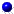Nonequispaced fast Fourier transform
Fast summation
Fast summation

# Fast summation based on NFFT

This library of C functions computes approximations of sums of the form $$f(y_j) := \sum\limits_{k=1}^N \alpha_k K(y_j-x_k)$$ $$(j =1,\dots,M)$$ based on the recently developed fast Fourier transform at nonequispaced knots (NFFT). Here $$K$$ are special kernels, e.g., $\frac{1}{x^2},\, \frac{1}{|x|}, \, \log |x|, \, x^2 \log |x|,\, \frac{1}{x},$ and in its multivariate generalization for RBFs $$\mathcal K$$. Our algorithm can be modified for other kernels frequently used in the approximation by RBFs, e.g., the Gaussian or the (inverse) multiquadric $$(x^2+c^2)^{\pm 1/2}$$.

New kernels are easily incorporated by defining an appropriate C-function (see kernels.c for some examples).

fastsum_test.c is an example for the usage of the library. The MATLAB script file fastsum_test.m calls the MATLAB function fastsum.m, which is a simple example for the usage in MATLAB. In summary it requires $$\mathcal O (N \log N +M)$$ arithmetic operations.

The algorithms are implemented by Markus Fenn in ./applications/fastsum. The OpenMP parallelization was implemented by Toni Volkmer. The Matlab interface was implemented by Michael Quellmalz in ./matlab/fastsum. The Julia interface was implemented by Michael Schmischke in ./julia/fastsum. Related paper arePotts, D. and Steidl, G.
Fast summation at nonequispaced knots by NFFTs.
SIAM J. on Sci. Comput. 24, 2013-2037. (full paper ps, pdf),  2004Potts, D., Steidl, G. and Nieslony, A.
Fast convolution with radial kernels at nonequispaced knots.
Numer. Math. 98, 329-351. (full paper ps, pdf),   2004Fenn, M. and Steidl, G.
Fast NFFT based summation of radial functions.
Sampling Theory in Signal and Image Processing 3/1, 1-28 (2004)Fenn, M. and Potts, D.
Fast summation based on fast trigonometric transforms at nonequispaced nodes.
Numer. Linear Algebra Appl. 12, 161-169. (full paper ps, pdf),   2005Pöplau, G., Potts, D. and van Rienen, U.
Calculation of 3D Space-Charge Fields of Bunches of Charged Particles by Fast Summation.
in: Proceedings of SCEE 2004 (5th International Workshop on Scientific Computing in Electrical Engineering), Springer-Verlag (full paper ps, pdf ),   2005